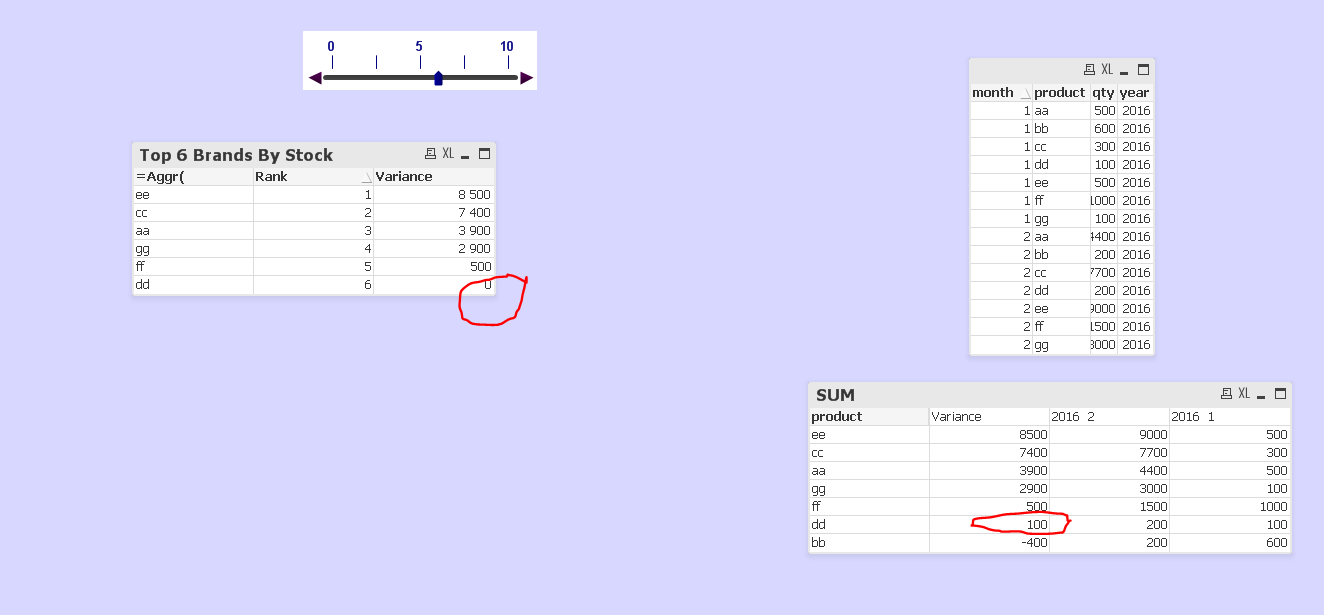Announcements
You can succeed best and quickest by helping others to succeed. Join the conversation.
cancel
Showing results for
Did you mean:Not applicable

## Calculate rank based on current month vs previous month

Greetings

I would like to rank the products based on the difference of current month and prior month.

(sum({<product= {"=rank(sum(qty), product<=\$(vRank)"}, year = 2016  , month = 2 >}  qty)  )

-

(sum({<product= {"=rank(sum(qty), product<=\$(vRank)"}, year = 2016  , month = 1 >}  qty)  )

Thanks!

1 Solution

Accepted SolutionsMVP

Your are still using the old  buggy expression, use

(sum({< year = {\$(vMaxYear)}, month= {\$(vMaxMth)}>} qty)  -
(
sum({< year = {\$(vMaxYear)}, month= {\$(vLstMth)}>} qty) )

10 RepliesMVP

Sorry, I don't understand.

This is not correct syntax:

{"=rank(sum(qty), product<=\$(vRank)"}

Maybe you want

{"=rank(sum(qty))<=\$(vRank)"}Not applicable
Author

Thanks for responding.

The rank must based on the difference of qty of Month 2 and 1.MVP

Maybe create a straight table with dimension product and as expression

=Rank(

(sum({< year = 2016  , month = 2 >}  qty)  )

-

(sum({< year = 2016  , month = 1 >}  qty)  )

)Not applicable
Author

Hi

It's working if the default selection is for all.

If I calculate for top N then not working.MVP

Not sure what you are telling me or what you are trying to do.

Could you upload a small sample QVW and describe based on your sample the requested result?Not applicable
Author

I would like to achieve the TOP 5 products based on the differences.

Please find attach the sample qvw file.MVP

Maybe like thisNot applicable
Author

If I will select top 6 then the result is showing incorrect.MVP

Your are still using the old  buggy expression, use

(sum({< year = {\$(vMaxYear)}, month= {\$(vMaxMth)}>} qty)  -
(
sum({< year = {\$(vMaxYear)}, month= {\$(vLstMth)}>} qty) )